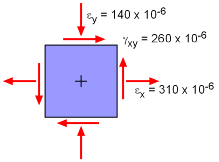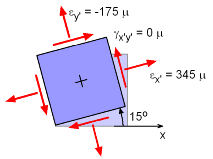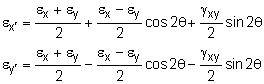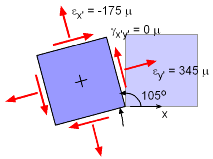Ch 8. Strain Analysis Multimedia Engineering Mechanics PlaneStrain Mohr's Circlefor Strain StrainGages
 Chapter 1. Stress/Strain 2. Torsion 3. Beam Shr/Moment 4. Beam Stresses 5. Beam Deflections 6. Beam-Advanced 7. Stress Analysis 8. Strain Analysis 9. Columns Appendix Basic Math Units Basic Equations Sections Material Properties Structural Shapes Beam Equations Search eBooks Dynamics Fluids Math Mechanics Statics Thermodynamics Author(s): Kurt Gramoll ©Kurt GramollMECHANICS - EXAMPLEStrain Element Example What is the largest principle strain for the strain element shown on the left? Solution The given strain components are,        εx = 310×10-6 = 310 μ      εy = -140×10-6 = -140 μ      γxy = 260×10-6 = 260 μ where m represents micro-strains. The principal strain equations could be used directly, but another way is to find the principal angles, and then use them to find the principal strains. The principle angles for this strain state are      tan 2θp =  γxy / (εx - εy)                 = 260/(310 + 140) = 0.5778      θp = (tan-1 0.5778) / 2      θp = 15.0°, 105.0°Rotated Strain Element, 15° Notice there are two possibilities. Both will give the same principal strains as will be shown below. For 15o, the two normal strains are,εx' = [310 + (-140)] / 2 + [310 - (-140) ] / 2 cos30               + 260/2 sin30          = 85 + 194.9 + 65.0 = 344.9 μ      εy' = [310 + (-140)] / 2 - [310 - (-140)] / 2 cos30              - 260 / 2 sin30          = 85 - 194.9 - 65 = -174.9 μ The principal strains are,      ε1 = εx' = 344.9 μ @ 15°      ε2 = εy' = -174.9 μ @ 15°Rotated Strain Element, 105° The other angle, 105°, will give the same results, but x' and y' will be reversed.εx' = [310 + (-140)] / 2 + [310 - (-140)] / 2 cos210               + 260/2 sin210          = 85 - 194.9 - 65 = -174.9 μ      εy' = [310 + (-140)] / 2 - [310 - (-140)] / 2 cos210              - 260/2 sin210          = 85 + 194.9 + 65.0 = 344.9 μ The principal strains are,      ε1 = εy' = 344.9 μ @ 105°      ε2 = εx' = -174.9 μ @ 105° As expected, the principal strain values are the same, the new coordinate, x' and y' are just reversed. But actually, the direction are the same sine this angle is 90 degree off from the previous angle.

Practice Homework and Test problems now available in the 'Eng Mechanics' mobile app
Includes over 400 problems with complete detailed solutions.
Available now at the Google Play Store and Apple App Store.